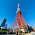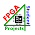# How to Read Image in VHDL

## This VHDL tutorial is to tell you how to read images in VHDL in a way that the images can be loaded into the block memory of the FPGA during synthesis or simulation.Since VHDL cannot read image files such as BMP, JPG, TIF, etc. directly, images are required to be converted into binary text files so that VHDL can read them using the TEXTIO VHDL package.
To convert images into binary text files, you can use Matlab or C. Once the image binary text files are ready, you can copy it to the project folder.

### Then, in VHDL, the binary text files can be read using the following function:

```-- by FPGA4student.com
impure function init_mem(mif_file_name : in string) return mem_type is
file mif_file : text open read_mode is mif_file_name;
variable mif_line : line;
variable temp_bv : bit_vector(DATA_WIDTH-1 downto 0);
variable temp_mem : mem_type;
begin
for i in mem_type'range loop
temp_mem(i) := to_stdlogicvector(temp_bv);
end loop;
return temp_mem;
end function;
```
It is noted that you need to include the TEXTIO package in the VHDL code by "use std.textio.all". Then, to load the image data in the binary text files into the block memory, you just need to add the following line to the VHDL code:
```signal ram_block: mem_type := init_mem(IMAGE_FILE_NAME);
```
Now, let's do an example code for reading images in VHDL. For simplicity, let's assume that the following is the content of the binary text file that we converted from a gray image.
```00001111
11100000
00000011
10101010
00110011
11001100
11101110
00000000
00001111
11110000
11000011
00000100
11111000
10001000
01111000
10001010
```
Then, save the image binary file as "IMAGE_FILE.MIF" and put it to the project folder. Now, write a VHDL code to read this image binary text file and initialize it into a block memory during synthesis or simulation.

### Below is the VHDL code for reading image files into FPGA. The code is synthesizable.

```library ieee;
use ieee.std_logic_1164.ALL;
use ieee.numeric_std.ALL;
use std.textio.all;
-- FPGA4student.com: FPGA/Verilog/VHDL projects for students
-- VHDL tutorial: How to Read images in VHDL
generic (
DATA_WIDTH     : integer := 8;
IMAGE_SIZE  : integer := 15;
IMAGE_FILE_NAME : string :="IMAGE_FILE.MIF"
);
port(
clock: IN STD_LOGIC;
data: IN std_logic_vector ((DATA_WIDTH-1) DOWNTO 0);
we: IN STD_LOGIC;
re: IN STD_LOGIC;
q: OUT std_logic_vector ((DATA_WIDTH-1) DOWNTO 0));

TYPE mem_type IS ARRAY(0 TO IMAGE_SIZE) OF std_logic_vector((DATA_WIDTH-1) DOWNTO 0);

impure function init_mem(mif_file_name : in string) return mem_type is
file mif_file : text open read_mode is mif_file_name;
variable mif_line : line;
variable temp_bv : bit_vector(DATA_WIDTH-1 downto 0);
variable temp_mem : mem_type;
begin
for i in mem_type'range loop
temp_mem(i) := to_stdlogicvector(temp_bv);
end loop;
return temp_mem;
end function;

signal ram_block: mem_type := init_mem(IMAGE_FILE_NAME);

begin
process (clock)
begin
if (rising_edge(clock)) then
if (we = '1') then
end if;
if (re = '1') then
end if;
end if;
end process;

end behavioral;
```

#### To verify if the image binary text file is read properly, let's write a testbench to test it in a simulation. Following is the testbench for reading image in VHDL:

```LIBRARY ieee;
USE ieee.std_logic_1164.ALL;
use ieee.numeric_std.all;
-- FPGA4student.com: FPGA/Verilog/VHDL projects for students
-- VHDL tutorial: How to Read images in VHDL
PORT(
clock : IN  std_logic;
data : IN  std_logic_vector(7 downto 0);
rdaddress : IN  std_logic_vector(3 downto 0);
wraddress : IN  std_logic_vector(3 downto 0);
we : IN  std_logic;
re : IN  std_logic;
q : OUT  std_logic_vector(7 downto 0)
);
END COMPONENT;
--Inputs
signal clock : std_logic := '0';
signal data : std_logic_vector(7 downto 0) := (others => '0');
signal rdaddress : std_logic_vector(3 downto 0) := (others => '0');
signal wraddress : std_logic_vector(3 downto 0) := (others => '0');
signal we : std_logic := '0';
signal re : std_logic := '0';
--Outputs
signal q : std_logic_vector(7 downto 0);

-- Clock period definitions
constant clock_period : time := 10 ns;```
```   signal i: integer;
BEGIN
clock => clock,
data => data,
we => we,
re => re,
q => q
);

-- Clock process definitions
clock_process :process
begin
clock <= '0';
wait for clock_period/2;
clock <= '1';
wait for clock_period/2;
end process;
-- Stimulus process
stim_proc: process
begin
data <= x"00";
we <= '0';
re <= '0';
wait for 100 ns;
re <= '1';
for i in 0 to 15 loop
wait for 20 ns;
end loop;
wait;
end process;

END;```
Now, it's time to run the simulation and check if the image binary text file is loaded correctly into the block memory. The following figure shows that the image was read properly into the block RAM of FPGAs.To read the image data in the block memory for testing your image processing design, just provide read addresses and enable memory reading. The following simulation waveform shows the image data in the binary text file is read out correctly at the output port of the block RAM.1.1.